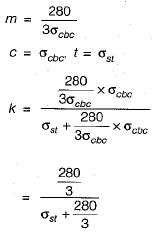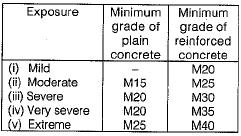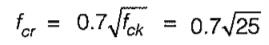Courses

# Test: Miscellaneous - 1

## 10 Questions MCQ Test GATE Civil Engineering (CE) 2022 Mock Test Series | Test: Miscellaneous - 1

Description
This mock test of Test: Miscellaneous - 1 for Civil Engineering (CE) helps you for every Civil Engineering (CE) entrance exam. This contains 10 Multiple Choice Questions for Civil Engineering (CE) Test: Miscellaneous - 1 (mcq) to study with solutions a complete question bank. The solved questions answers in this Test: Miscellaneous - 1 quiz give you a good mix of easy questions and tough questions. Civil Engineering (CE) students definitely take this Test: Miscellaneous - 1 exercise for a better result in the exam. You can find other Test: Miscellaneous - 1 extra questions, long questions & short questions for Civil Engineering (CE) on EduRev as well by searching above.
QUESTION: 1

### The relation between modulus of rupture fcr, splitting strength fcs and direct tensile strength fct is given by

Solution:

The approximate relations are,
fcs  = 0.66 fcr
fct = 0.50 fcr

QUESTION: 2

### As compared to working stress method of design, limit state method takes concrete to

Solution:

The material factor of safety in limit state method is less as compared to that in working stress method.

QUESTION: 3

### If the depth of neutral axis for a singly reinforced balanced rectangular section is represented by kd in working stress design, then the value of k for a balanced section,

Solution:

The value of k is given by,
k = mc/(t + mc)
For a balanced section,QUESTION: 4

Examine the following statements:
1. Factor of safety for steel should be based on its yield stress.
2. Factor of safety for steel should be based on its ultimate stress.
3. Factor of safety for concrete should be based on its yield stress.
4. Factor of safety for concrete should be based on its ultimate stress.
The correct statements are:

Solution:

A beam collapses when stress is equal to its yield strength and stress in concrete reaches its ultimate strength.

QUESTION: 5

What should be the minimum grade of reinforced concrete in and around sea coast construction?

Solution:Concrete in sea water or exposed directly along the sea coast shall be at least M20 grade in the case of plain concrete and M30 in case of reinforced concrete. The use of slag or pozzolana cement is advantageous under such conditions.

QUESTION: 6

On which one of the following concepts is the basic principle of structural design based?

Solution:

In tall, buildings, it is important to control lateral displacements within the serviceability limit state. A structural system may be classified as follows:
(i) Buildings frame system
(ii) Moment resisting frame system
(iii) Dual frame system
(iv) Tube system
In a moment resistant frame, the relative stiffness of beam and columns is very important. A frame may be designed using weak column-strong beam proportions or strong column - weak beam proportions. A frame with weak beam-strong column is more stable and therefore highly desirable.

QUESTION: 7

When is a masonry wall known as a shear wall?

Solution:

A wall which carries horizontal loads in its own plane is called shear wall.

QUESTION: 8

A masonry wall has height ‘h' ,length L and thickness 't'. The allowable stress based or slenderness is calculated on the basis of

Solution:

The slenderness ratio of a wall or column is defined as the ratio of effective height to its effective thickness. For a wall, the effective height is replaced by the effective length if effective height happens to be less.

QUESTION: 9

As the span of a bridge increases, how does the impact factor vary?

Solution:

Impact factor for RC bridges, I = 4.5/6 + L
where L is the length of span in meters.

QUESTION: 10

At what stress does the first flexural crack appear in RCC beams made of M25 grade concrete?

Solution:

Flexural strength of concrete is given by,= 0.7 x 5 = 3.5 MPa